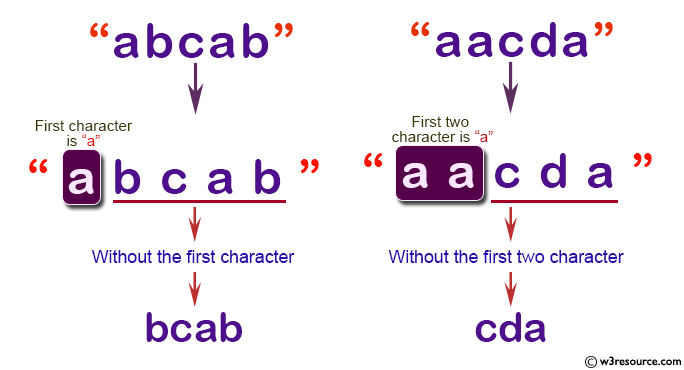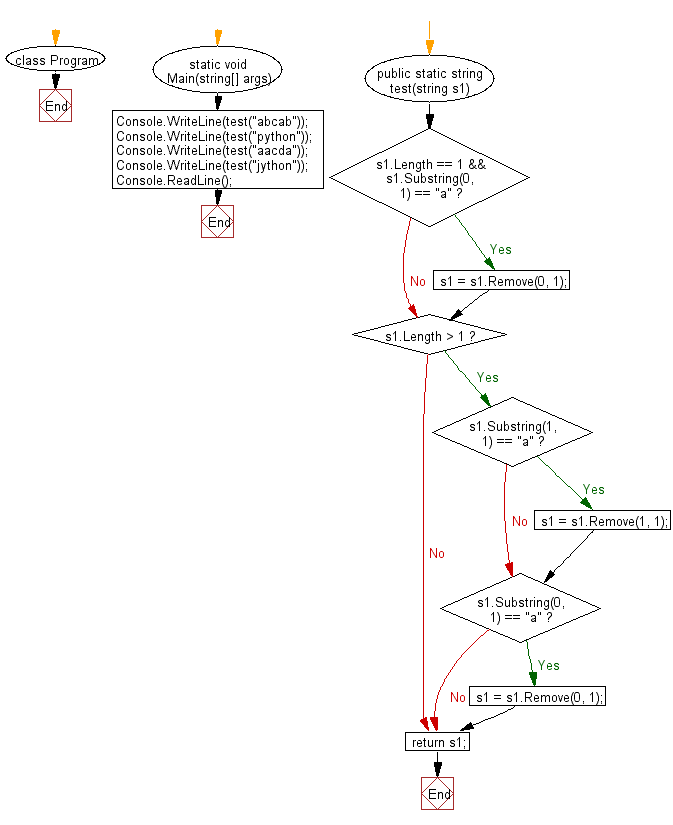﻿ C# - Return the string without the first or first two is a# C# Sharp Basic Algorithm Exercises: Create a new string from a given string. If the first or first two characters is 'a', return the string without those 'a' otherwise return the original given string

## C# Sharp Basic Algorithm: Exercise-87 with Solution

Write a C# Sharp program to create a new string from a given string. If the first or first two characters are 'a', return the string without those 'a' otherwise return the original given string.

Pictorial Presentation:Sample Solution:-

C# Sharp Code:

``````using System;
namespace exercises
{
class Program
{
static void Main(string[] args)
{
Console.WriteLine(test("abcab"));
Console.WriteLine(test("python"));
Console.WriteLine(test("aacda"));
Console.WriteLine(test("jython"));

}

public static string test(string s1)
{
if (s1.Length == 1 && s1.Substring(0, 1) == "a")
{
s1 = s1.Remove(0, 1);
}

if (s1.Length > 1)
{
if (s1.Substring(1, 1) == "a")
{
s1 = s1.Remove(1, 1);
}

if (s1.Substring(0, 1) == "a")
{
s1 = s1.Remove(0, 1);
}
}

return s1;
}
}
}
```
```

Sample Output:

```bcab
python
cda
jython
```

Flowchart:C# Sharp Code Editor:

Improve this sample solution and post your code through Disqus

What is the difficulty level of this exercise?

Test your Programming skills with w3resource's quiz.

﻿